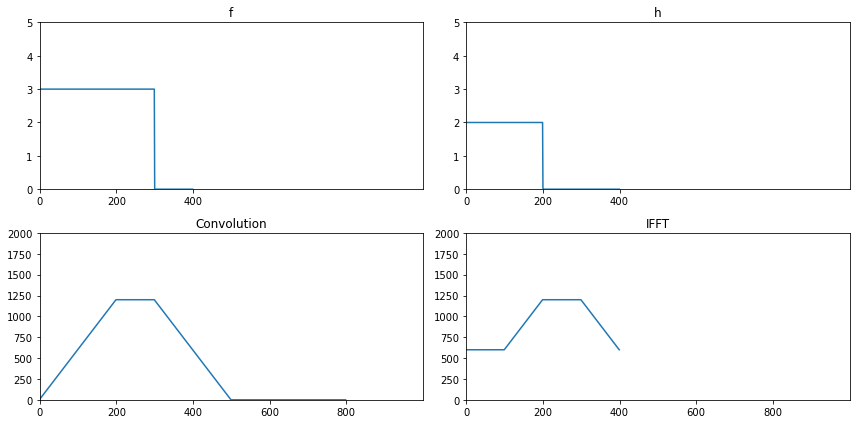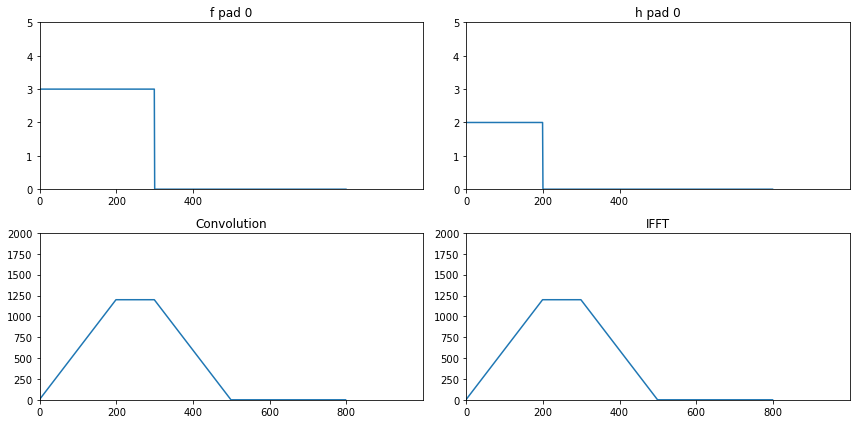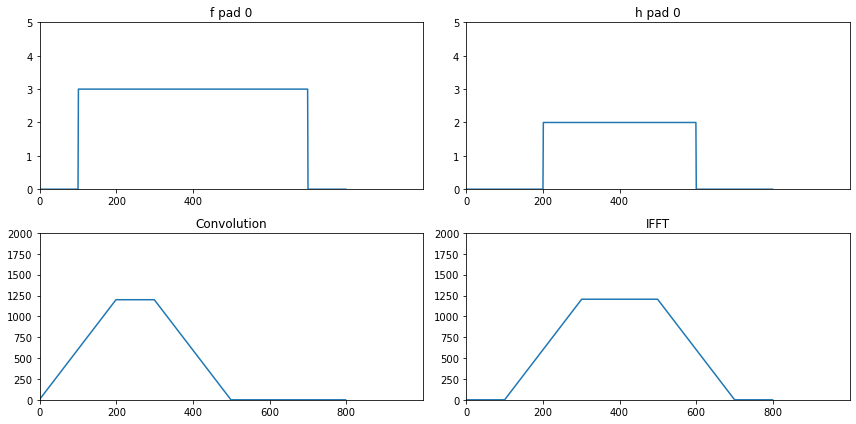• ## 二维DFT

千次阅读 2014-11-17 15:43:04
学习DIP第4天 傅里叶变换数学原理会在后续完整介绍，目前只实现代码，观察下结果，公式在上一篇博客中已经描述  内容迁移至 http://www.face2ai.com/DIP-2-2-二维...http://www.tony4ai.com/DIP-2-2-二维DFT/...
学习DIP第4天傅里叶变换数学原理会在后续完整介绍，目前只实现代码，观察下结果，公式在上一篇博客中已经描述

内容迁移至

http://www.face2ai.com/DIP-2-2-二维DFT/

http://www.tony4ai.com/DIP-2-2-二维DFT/
展开全文数字图像处理 离散傅里叶变换 c语言
• 如何把上面的结果推广到二维的情形，是一个值得研究的问题。一个矩阵的2-D（2维）DFT通常用行列法化为1-DDFT来计算。但是，用行列法把上述一维的结果推广到二维是困难的。作者得到了计算二维情形的一些新的直接公式...
• 本篇直接对图片使用二维DFT公式，意在与两次一维DFT叠加的方式做对比。 二维DFT公式： X[k]=∑n=0N−1x[n]e−j2πNkn X[k]=\sum_{n=0}^{N-1}x[n]e^{-j\frac{2\pi}{N}kn} X[k]=n=0∑N−1​x[n]e−jN2π​kn %% Q1 2D...
本篇直接对图片使用二维DFT公式，意在与两次一维DFT叠加的方式做对比。
二维DFT公式：
$F(u,v)=\sum_{x=0}^{M-1}\sum_{y=0}^{N-1}f(x,y)e^{-j2\pi(\frac{ux}{M}+\frac{vy}{N})}$
MATLAB代码
直接用四层循环嵌套实现公式的计算过程
%% Q1 2D-DFT
image=im2double(rgb2gray(raw_image));
[M,N]=size(image); % get the image size
T=zeros(M,N);
tic % start counting
for x = 1:M % for every row
for y = 1:N % for every column
for u =1:M
for v = 1:N
E = exp(-1i*2*pi*(((u-1)*(x-1)/M)+((v-1)*(y-1)/N))); % get exp kernel
T(x,y) = T(x,y) + image(u,v)*E;
end
end
end
end
toc % stop counting
subplot(1,2,1),imshow(raw_image),title('raw');
subplot(1,2,2),imshow(T),title('2D-DFT');

用我最喜欢的憨憨龟来测试用时1081.484753 秒，可以说是最憨的一种实现方式了。
另一篇文章介绍了一维的DFT叠加方式实现。


展开全文matlab 信号处理
• 用快速多项式变换_FPT_计算二维DFT的混合算法
• 目录二维DFT和IDFT的一些性质二维离散卷积定理二维离散傅里叶变换性质的小结 二维DFT和IDFT的一些性质 二维离散卷积定理 二维循环卷积表达式： (f⋆h)(x,y)=∑m=0M−1∑n=0N−1f(m,n)h(x−m,y−n)(4.94)(f \star h)...
目录二维DFT和IDFT的一些性质二维离散卷积定理二维离散傅里叶变换性质的小结
二维DFT和IDFT的一些性质
二维离散卷积定理
二维循环卷积表达式：
$(f \star h)(x, y) = \sum_{m=0}^{M-1} \sum_{n=0}^{N-1} f(m,n)h(x-m, y-n) \tag{4.94}$
二维卷积定理为：
$(f \star h)(x, y) \Leftrightarrow (F\bullet H)(u, v) \tag{4.95}$
$(f \bullet h)(x, y) \Leftrightarrow \frac{1}{MN}(F\star H)(u, v) \tag{4.96}$
$f$和$h$的空间卷积的傅里叶变换，是它们的变换的乘积。
空间卷积是空间域滤波的基础，式(4.95)是在空间域和频率域滤波之间建立等价关系的纽带。
# 一维卷积与一维傅里叶变换
f = np.zeros()
f[:300] = 3

h = np.zeros([f.shape])
h[:200] = 2

# 卷积
F_con = np.convolve(f, h)

# 傅里叶变换，未填充零的结果
f_fft = np.fft.fft(f)
f_fft = np.fft.fftshift(f_fft)
h_fft = np.fft.fft(h)
h_fft = np.fft.fftshift(h_fft)

fh_fft = f_fft * h_fft

# 傅里叶反变换
fh_ifft = np.abs(np.fft.ifft(fh_fft))

fig = plt.figure(figsize=(12, 6))
plt.subplot(2, 2, 1), plt.plot(f), plt.ylim([0, 5]), plt.xlim([0, 1000]), plt.xticks([0, 200, 400]), plt.title('f')
plt.subplot(2, 2, 2), plt.plot(h), plt.ylim([0, 5]), plt.xlim([0, 1000]), plt.xticks([0, 200, 400]), plt.title('h')
plt.subplot(2, 2, 3), plt.plot(F_con), plt.ylim([0, 2000]), plt.xlim([0, 1000]), plt.xticks([0, 200, 400, 600, 800]),
plt.title('Convolution')
plt.subplot(2, 2, 4), plt.plot(fh_ifft), plt.ylim([0, 2000]), plt.xlim([0, 1000]), plt.xticks([0, 200, 400, 600, 800]),
plt.title('IFFT')
plt.tight_layout()
plt.show()从上图可以看出，卷积与傅里叶变换的结果不一样，这种错误可以通过对原函数填充零来纠正。要使得它们的长度$P$相同，则有
$P \ge A + B - 1 \tag{4.97}$
从下面的结果可以看出，直接的零填充得到的结果跟卷积是一样的。镜像和复制的填充结果不太一样。
二维填充的公式：
$f_P(x, y) = \begin{cases} f(x, y), & 0 \leq x \leq A -1和 0 \leq y \leq B-1 \\ 0, & A \leq x \leq P 或 B \leq y \leq Q \end{cases} \tag{4.98}$
$h_P(x, y) = \begin{cases} h(x, y), & 0 \leq x \leq C -1和 0 \leq y \leq D-1 \\ 0, & C \leq x \leq P 或 D \leq y \leq Q \end{cases} \tag{4.99}$
$P \ge A + C - 1 \tag{4.100}$
$Q \ge B + D - 1 \tag{4.101}$
DFT算法通过偶数大小的阵列时速度通常更快，因此经常选择$P$和$Q$的最小偶数整数。
如果两个阵列大小相同，则意味着
$P = 2M \tag{4.102}$
$Q = 2N \tag{4.103}$
在两个函数中，如果有一个或两个函数的值在取样区间的末尾不是0，那么把0添加到函数中来消除交叠错误时，会导致函数不连续。这类似于用一个盒式函数来乘以一下函数，在频率域是这一相乘意味着原变换与一个$\text{sinc}$函数的卷积，进行导致由$\text{sinc}$函数的高频分量产生所谓的频率泄露。
频率泄露会使得图像块效应。
开窗或切趾法

降低工频率泄漏的方法，就是让取样后的函数乘以另一个两端平滑地过渡到0的函数(窗函数)

# 傅里叶变换，填充堆的结果
f_fft = np.fft.fftshift(f_fft)
h_fft = np.fft.fftshift(h_fft)

fh_fft = f_fft * h_fft

# 傅里叶反变换
fh_ifft = np.abs(np.fft.ifft(fh_fft))

fig = plt.figure(figsize=(12, 6))
plt.subplot(2, 2, 1), plt.plot(f_pad), plt.ylim([0, 5]), plt.xlim([0, 1000]), plt.xticks([0, 200, 400]), plt.title('f pad 0')
plt.subplot(2, 2, 2), plt.plot(h_pad), plt.ylim([0, 5]), plt.xlim([0, 1000]), plt.xticks([0, 200, 400]), plt.title('h pad 0')
plt.subplot(2, 2, 3), plt.plot(F_con), plt.ylim([0, 2000]), plt.xlim([0, 1000]), plt.xticks([0, 200, 400, 600, 800]),
plt.title('Convolution')
plt.subplot(2, 2, 4), plt.plot(fh_ifft), plt.ylim([0, 2000]), plt.xlim([0, 1000]), plt.xticks([0, 200, 400, 600, 800]),
plt.title('IFFT')
plt.tight_layout()
plt.show()# 傅里叶变换，镜像

f_fft = np.fft.fftshift(f_fft)
h_fft = np.fft.fftshift(h_fft)

fh_fft = f_fft * h_fft

# 傅里叶反变换
fh_ifft = np.abs(np.fft.ifft(fh_fft))
fh_ifft = 2394 - fh_ifft
fig = plt.figure(figsize=(12, 6))
plt.subplot(2, 2, 1), plt.plot(f_pad), plt.ylim([0, 5]), plt.xlim([0, 1000]), plt.xticks([0, 200, 400]), plt.title('f pad 0')
plt.subplot(2, 2, 2), plt.plot(h_pad), plt.ylim([0, 5]), plt.xlim([0, 1000]), plt.xticks([0, 200, 400]), plt.title('h pad 0')
plt.subplot(2, 2, 3), plt.plot(F_con), plt.ylim([0, 2000]), plt.xlim([0, 1000]), plt.xticks([0, 200, 400, 600, 800]),
plt.title('Convolution')
plt.subplot(2, 2, 4), plt.plot(fh_ifft), plt.ylim([0, 2000]), plt.xlim([0, 1000]), plt.xticks([0, 200, 400, 600, 800]),
plt.title('IFFT')
plt.tight_layout()
plt.show()二维离散傅里叶变换性质的小结

名称
表达式

$f(x, y)$的DFT
$F(u, v) = \sum_{x = 0}^{M - 1} \sum_{y = 0}^{N - 1} f(x, y) e^{-j2\pi(u x/M + v y /N)}$

$F(u, v)$的IDFT
$f(x, y) = \frac{1}{MN}\sum_{u = 0}^{M - 1} \sum_{v = 0}^{N - 1} F(u, v) e^{j2\pi(u x /M + vy /N)}$


展开全文图像处理 python 卷积 numpy
• 在图像处理中，傅里叶变换应该是二维的，而且，是离散的，即：二维DFT。一个图像尺寸为的函数的离散傅里叶变换由以下等式给出：  (1) 像在一维中的一样，此表达式对u值（u=0,1,2,...,M-1）和v值(v=0,1,2,..,N-1...
转自：http://blog.csdn.net/carson2005/article/details/6586583
在图像处理中，傅里叶变换应该是二维的，而且，是离散的，即：二维DFT。一个图像尺寸为的函数的离散傅里叶变换由以下等式给出：(1)
像在一维中的一样，此表达式对u值（u=0,1,2,...,M-1）和v值(v=0,1,2,..,N-1)计算。给出,可以通过傅里叶反变换来计算得到f(x,y),即：（2）
其中，x=0,1,...,M-1,y=0,1,...,N-1;上面两个式子共同构成了二位离散傅里叶变换对（DFT）。变量u和v是变换或频率变量，x和y是图像变量。
幅度（频率谱）：（3）
相角：（4）
功率谱(谱密度)：（5）
其中，R(u,v)和I(u,v)分别是F(u,v)的实部和虚部。
通常，在傅里叶变换之前用乘以输入图像函数，由于指数的性质，可以看出：（6）
其中表示一种傅里叶变换。这个等式说明，傅里叶变换的原点（F（0,0））被设置在u=M/2和N/2上。换句话说，用乘以f(x,y)将F)(u,v)原点变换到（M/2,N/2）,它是二维离散傅里叶变换设置的区域的中心。我们将此频率域的范围指定为频率矩形，它从u=0到u=M-1,从v=0到v-N-1，其中，u和v都是整数，而且，为了确保移动后的坐标为整数，要求M和N都是偶数。
从（1）我们可以发现，(u,v)=(0,0)的变换值为：（7）
即f(x,y)的平均值，也就是说，如果f(x,y)是一幅图像，在原点的傅里叶变换等于图像的平均灰度值。因为在原点处常常为0，F（0,0）有时被称作频率谱的直流成分。

无法联系到原作者，请看到后联系hjwang1@163.com

展开全文• 在图像处理中，傅里叶变换应该是二维的，而且，是离散的，即：二维DFT。一个图像尺寸为的函数的离散傅里叶变换由以下等式给出：  (1) 像在一维中的一样，此表达式对u值（u=0,1,2,...,M-1）和v值(v=0,1,2,..,N-1)...
• 该算法通过导频位置偏移量预校正、二维离散 Fourier变换(DFT)和变换域滤波消除噪声的影响,利用变换域补零和二维反离散Fourier变换进行信道传输函数插值,并使用变形 cos窗抑制插值过程中的频谱扩散。仿真结果表明:该...
• 题目：用Matlab 实现以下3个信号的DFT 分析，具体要求：画出对应信号的时域波形及其振幅、相位谱。(画出物理坐标)Hzf t f A t f A t 50).2sin()3();2sin()2();()1(0202010=其中ππδ解答：代码如下：(1) N=64;%生成...
• 二维傅里叶变换的实部 R(u,v) 和 虚部 I(u,v) 是与 F(u,v) 大小相同的数组。因为 R 和 I 均可为正负，所以需要能够在整个 [-π ，π] 范围内计算反正切 MATLAB 中使用 函数 atan2 执行这一算法： phi = atan2(I...《数字图像处理》
• 目录二维DFT和IDFT的一些性质傅里叶频谱和相角 二维DFT和IDFT的一些性质 傅里叶频谱和相角 F(u,v)=R(u,v)+jI(u,v)=∣F(u,v)∣ejϕ(u,v)(4.86)F(u, v) = R(u, v) + jI(u, v) = |F(u, v)|e^{j\phi(u,v)} \tag{4.86}F(u...图像处理 python opencv numpy
• 图像的二维傅里叶变换的公式始终没成功推出来，执着地僵持了很久，于是成此文。 二维傅里叶变换的四对公式不清楚可以移步这篇博客的第4种case（因为脉冲阵列就是2维离散周期信号）。 首先应当明确数字图像（M行*N列...图像采样和量化
• 目录二维DFT和IDFT的一些性质空间间隔和频率间隔的关系平移和旋转周期性对称性 二维DFT和IDFT的一些性质 空间间隔和频率间隔的关系 Δu=1MΔT(4.69)\Delta u = \frac{1}{M \Delta T} \tag{4.69}Δu=MΔT1​(4.69) Δ...python 图像处理 numpy opencv
• **f=imread('4.3.tif'); F=fft2(f);** 当需要对图像进行填充时候 F=fft2(f,P,Q) %fft2讲M x N的图像填充为P x Q的图像 傅里叶频谱可以使用函数abs来获得 S=abs(F); %实部和虚部平方和的平方根 ...图像处理
• 3.2 在MATLAB中计算及观察二维DFTfunction t = func_3_2() %读取图片 f = imread('Fig0303(a).tif'); %返回傅里叶变换，大小为MxN,0填充 F = fft2(f); %计算每个元素的幅度 S = abs(F); %显示图片 imshow(S,[]) ......